# AS sequence

In an arithmetic sequence is given the difference d = -3 and a71 = 455.

a) Determine the value of a62
b) Determine the sum of 71 members.

a62 =  482
s71 =  39760

### Step-by-step explanation:

${s}_{71}=71\cdot \frac{{a}_{1}+{a}_{71}}{2}=71\cdot \frac{665+455}{2}=39760$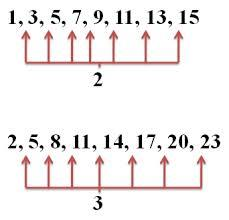Did you find an error or inaccuracy? Feel free to write us. Thank you!## Related math problems and questions:

• RemaindersIt is given a set of numbers { 170; 244; 299; 333; 351; 391; 423; 644 }. Divide this numbers by number 66 and determine set of remainders. As result write sum of this remainders.
• Chess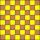How many ways can select 4 fields on a classic chessboard with 64 fields so that fields don't have the same color?
• SequenceBetween numbers 1 and 53 insert n members of the arithmetic sequence that its sum is 702.
• Sequence 3Write the first 5 members of an arithmetic sequence: a4=-35, a11=-105.
• Five element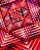The geometric sequence is given by quotient q = 1/2 and the sum of the first six members S6 = 63. Find the fifth element a5.
• GP - three membersThe second and third of a geometric progression are 24 and 12(c+1), respectively, given that the sum of the first three terms of progression is 76. determine the value of c.
• Sequence 2Write the first 5 members of an arithmetic sequence a11=-14, d=-1
• Geometric sequence 4It is given geometric sequence a3 = 7 and a12 = 3. Calculate s23 (= sum of the first 23 members of the sequence).
• Statistics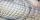The sum of all deviations from the arithmetic mean of the numerical sequence 4, 6, 51, 77, 90, 93, 95, 109, 113, 117 is:
• The sumThe sum of the first 10 members of the arithmetic sequence is 120. What will be the sum if the difference is reduced by 2?
• In the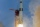In the arithmetic sequence a1 = 4.8, d = 0.4. How many consecutive members, starting with the first, need to be added so that the sum is greater than 170?
• Five membersWrite first 5 members geometric sequence and determine whether it is increasing or decreasing: a1 = 3 q = -2
• Sum of membersWhat is the sum of the first two members of the aritmetic progression if d = -4.3 and a3 = 7.5?
• Common differenceThe 4th term of an arithmetic progression is 6. If the sum of the 8th and 9th terms is -72, find the common difference.
• Determine AP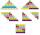Determine the difference of the arithmetic progression if a3 = 7, and a4 + a5 = 71
• RatiosReduce the numbers: 50 in a 1:2 ratio 111 at a ratio of 2:3 70 at 10:50 560 at a ratio of 3:8
• City market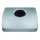Apples were sold at contractual prices at the city market. Calculate the average price of 1 kg of apples sold: Stand, Sale kg, Price CZK/kg 1 325 6.20 2 194 7.00 3 258 6.50 4 870 5.90 5 275 6.40